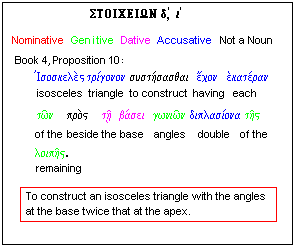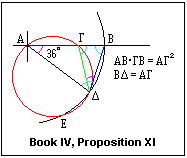## Lesson ιε': A Remarkable Proposition

Inscribing a pentagon in a circle is one of the most remarkable results in the early part of the ElementsIn Book IV, Proposition 11, Euclid shows how to inscribe a regular pentagon in a circle. This is the culmination of a long path beginning with Book II, Proposition 11, where it is shown how to divide a line segment AB into two parts, AΓ and ΓB, such that AB x ΓB = AΓ2. In Book IV, Proposition 10, this result is used to show how to construct an isosceles triangle with the equal angles at the base twice the angle at the apex. After this, Proposition 11 can then be demonstrated. Proposition 10 is an excellent exercise. If you can follow it, you can consider yourself a true Euclidean.

In a regular pentagon, each side subtends an angle of 360°/5 = 72° at the centre of the circumscribed circle, and we know from trigonometry that the ratio of the length of a side to the radius of the circumscribed circle is 2 sin (72°/2) = 1.1756. Given a circle, we can compute the length of the side, lay it off with a scale, and place it in the circle, easily constructing the pentagon. Alternatively, we could lay out a sector with a central angle of 72°, and draw the pentagon as easily. From a practical viewpoint, the task is not a difficult one, provided we have an accurate scale or an accurate protractor. Euclid shows that it can be done without these aids, merely with compass and straightedge.Book II, Proposition 11 shows how to construct a side of the pentagon (AΓ) starting with the radius of the circumscribed circle (AB). This side is placed in the circle, and the pentagon constructed, exactly as we would proceed in the last paragraph. The proposition shows, however, that this procedure gives the correct result. The proof is quite ingenious, and not at all obvious. In the Figure, AB is the given radius of the circle, and Γ is the point that divides it in the desired ratio, determined by Book II, Proposition 11 (or by calculation). A distance equal to AΓ is laid off from B to an intersection with a circle of radius AB and centre A. (These equal distances are shown in blue). A circle is circumscribed about the triangle, and the relation AB x ΓB = AΓ2 = ΔB2 is used to show that BΔ is tangent to the circumscribed circle, of which AB is a secant. This is the key to the proof.

We now need to show that triangle ΔΓB is isosceles, that the green side equals the blue side. This is done by noting that the angle in the chord ΔΓ, angle BΔΓ, equals the angle standing on the chord, angle ΓAΔ. The angle ABΔ = angle AΔB because AB = AΔ. Now, in the triangle ΔΓB, the two angles at B and Δ are equal to the two angles in triangle ABΔ at A and Δ. The third angles in the triangles are those at B and Γ, which must be equal, since the sum of the angles is 180° in either case. This proves that triangle ΔBΓ is isosceles. Therefore, ΓΔ = ΔB = AΓ, and triangle AΓΔ is also isosceles. Finally, we can use the fact that the exterior angle BΓΔ is the sum of the two opposite interior angles, which are equal from the equality of the sides that we have just proved, so that the angles at B and Δ are each twice the angle at A, so the angle at A is 36°, which was what was to be shown.